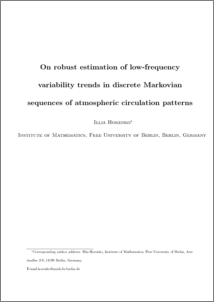Repository: Freie Universität Berlin, Math Department

# On robust estimation of low-frequency variability trends in discrete Markovian sequences of atmospheric circulation patterns

Horenko, I. (2008) On robust estimation of low-frequency variability trends in discrete Markovian sequences of atmospheric circulation patterns. J. Atm. Sci. . (In Press)Preview
PDF - Accepted Version
941kB

## Abstract

Identiﬁcation and analysis of temporal trends and low-frequency variability in discrete time series is an important practical topic in understanding and predic- tion of many atmospheric processes, for example, in analysis of climate change. Widely used numerical techniques of trend identiﬁcation (like local Gaussian ker- nel smoothing) impose some strong mathematical assumptions on the analyzed data and are not robust to model sensitivity. The latter becomes crucial when analyzing historical observation data with a short record. Two global robust nu- merical methods for the trend estimation in discrete non-stationary Markovian data based on diﬀerent sets of implicit mathematical assumptions are introduced and compared here. The methods are ﬁrst compared on a simple model exam- ple, the importance of mathematical assumptions on the data is explained and numerical problems of local Gaussian kernel smoothing are demonstrated. Pre- sented methods are applied to analysis of the historical sequence of atmospheric circulation patterns over UK between 1946-2007. It is demonstrated that the inﬂuence of the seasonal pattern variability on transition processes is dominated by the long-term eﬀects revealed by the introduced methods. Despite of the dif- ferences in the mathematical assumptions implied by both presented methods, almost identical symmetrical changes of the cyclonic and anticyclonic pattern probabilities are identiﬁed in the analyzed data, with the conﬁdence intervals being smaller then in the case of the local Gaussian kernel smoothing algorithm. Analysis results are investigated with respect to model sensitivity and compared to standard analysis technique based on a local Gaussian kernel smoothing. Finally, the implications of the discussed strategies on long-range predictability of the data-ﬁtted Markovian models are discussed.

Item Type: Article Mathematical and Computer Sciences > Mathematics > Applied Mathematics Department of Mathematics and Computer Science > Institute of Mathematics > BioComputing Group 180 Lars Putzig 06 Mar 2009 09:37 03 Mar 2017 14:40

Repository Staff Only: item control page